Open in App
Not now

# Detect and Remove the Outliers using Python

• Difficulty Level : Easy
• Last Updated : 10 Aug, 2021

An Outlier is a data-item/object that deviates significantly from the rest of the (so-called normal)objects. They can be caused by measurement or execution errors. The analysis for outlier detection is referred to as outlier mining. There are many ways to detect the outliers, and the removal process is the data frame same as removing a data item from the panda’s data frame.

Here pandas data frame is used for a more realistic approach as in real-world project need to detect the outliers arouse during the data analysis step, the same approach can be used on lists and series-type objects.

### Dataset:

Dataset used is Boston Housing dataset as it is preloaded in the sklearn library.

## Python3

 `# Importing``import` `sklearn``from` `sklearn.datasets ``import` `load_boston``import` `pandas as pd``import` `matplotlib.pyplot as plt` `# Load the dataset``bos_hou ``=` `load_boston()` `# Create the dataframe``column_name ``=` `bos_hou.feature_names``df_boston ``=` `pd.DataFrame(bos_hou.data)``df_boston.columns ``=` `column_name``df_boston.head()`

Output: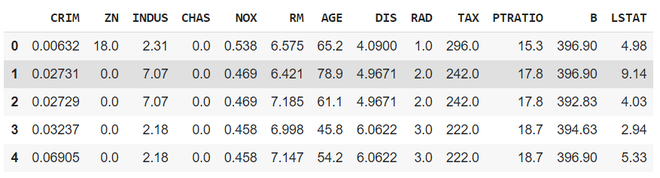part of the dataset

## Detecting the outliers

Outliers can be detected using visualization, implementing mathematical formulas on the dataset, or using the statistical approach. All of these are discussed below.

### 1. Visualization

Example 1: Using Box Plot

It captures the summary of the data effectively and efficiently with only a simple box and whiskers. Boxplot summarizes sample data using 25th, 50th, and 75th percentiles. One can just get insights(quartiles, median, and outliers) into the dataset by just looking at its boxplot.

## Python3

 `# Box Plot``import` `seaborn as sns``sns.boxplot(df_boston[``'DIS'``])`

Output: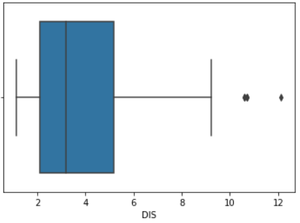Boxplot- DIS column

In the above graph, can clearly see that values above 10 are acting as the outliers.

## Python3

 `# Position of the Outlier``print``(np.where(df_boston[``'DIS'``]>``10``))`

Output: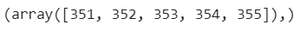Outlier’s Index

Example 2: Using ScatterPlot.

It is used when you have paired numerical data, or when your dependent variable has multiple values for each reading independent variable, or when trying to determine the relationship between the two variables. In the process of utilizing the scatter plot, one can also use it for outlier detection.

To plot the scatter plot one requires two variables that are somehow related to each other. So here, ‘Proportion of non-retail business acres per town’ and ‘Full-value property-tax rate per \$10,000’ are used whose column names are “INDUS” and “TAX” respectively.

## Python3

 `# Scatter plot``fig, ax ``=` `plt.subplots(figsize ``=` `(``18``,``10``))``ax.scatter(df_boston[``'INDUS'``], df_boston[``'TAX'``])` `# x-axis label``ax.set_xlabel(``'(Proportion non-retail business acres)/(town)'``)` `# y-axis label``ax.set_ylabel(``'(Full-value property-tax rate)/( \$10,000)'``)``plt.show()`

Output: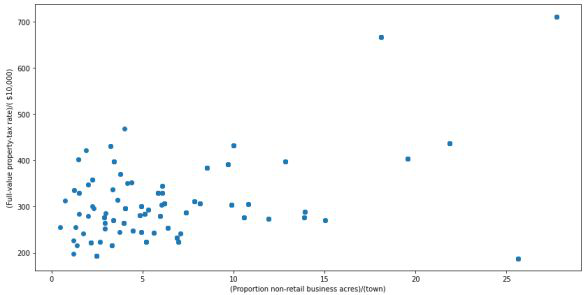Scatter Plot

Looking at the graph can summarize that most of the data points are in the bottom left corner of the graph but there are few points that are exactly;y opposite that is the top right corner of the graph. Those points in the top right corner can be regarded as Outliers.

Using approximation can say all those data points that are x>20 and y>600 are outliers. The following code can fetch the exact position of all those points that satisfy these conditions.

## Python3

 `# Position of the Outlier``print``(np.where((df_boston[``'INDUS'``]>``20``) & (df_boston[``'TAX'``]>``600``)))`

Output: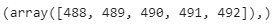Outlier’s Index

### 2. Z-score

Z- Score is also called a standard score. This value/score helps to understand that how far is the data point from the mean. And after setting up a threshold value one can utilize z score values of data points to define the outliers.

Zscore = (data_point -mean) / std. deviation

## Python3

 `# Z score``from` `scipy ``import` `stats``import` `numpy as np` `z ``=` `np.``abs``(stats.zscore(df_boston[``'DIS'``]))``print``(z)`

Output: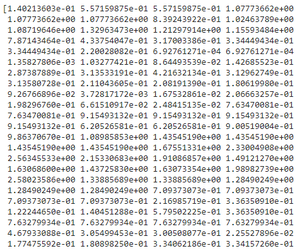part of the list(z)

The above output is just a snapshot of part of the data; the actual length of the list(z) is 506 that is the number of rows. It prints the z-score values of each data item of the column

Now to define an outlier threshold value is chosen which is generally 3.0. As 99.7% of the data points lie between +/- 3 standard deviation (using Gaussian Distribution approach).

## Python3

 `threshold ``=` `3` `# Position of the outlier``print``(np.where(z > ``3``))`

Output: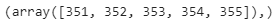Outlier’s Index

### 3. IQR (Inter Quartile Range)

IQR (Inter Quartile Range) Inter Quartile Range approach to finding the outliers is the most commonly used and most trusted approach used in the research field.

IQR = Quartile3 – Quartile1

## Python3

 `# IQR``Q1 ``=` `np.percentile(df_boston[``'DIS'``], ``25``,``                   ``interpolation ``=` `'midpoint'``)` `Q3 ``=` `np.percentile(df_boston[``'DIS'``], ``75``,``                   ``interpolation ``=` `'midpoint'``)``IQR ``=` `Q3 ``-` `Q1`

Output: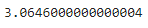To define the outlier base value is defined above and below datasets normal range namely Upper and Lower bounds, define the upper and the lower bound (1.5*IQR value is considered) :

upper = Q3 +1.5*IQR

lower = Q1 – 1.5*IQR

In the above formula as according to statistics, the 0.5 scale-up of IQR (new_IQR = IQR + 0.5*IQR) is taken, to consider all the data between 2.7 standard deviations in the Gaussian Distribution.

## Python3

 `# Above Upper bound``upper ``=` `df_boston[``'DIS'``] >``=` `(Q3``+``1.5``*``IQR)` `print``(``"Upper bound:"``,upper)``print``(np.where(upper))` `# Below Lower bound``lower ``=` `df_boston[``'DIS'``] <``=` `(Q1``-``1.5``*``IQR)``print``(``"Lower bound:"``, lower)``print``(np.where(lower))`

Output: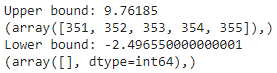Defined bounds and outlier’s index respective to the bounds

## Removing the outliers

For removing the outlier, one must follow the same process of removing an entry from the dataset using its exact position in the dataset because in all the above methods of detecting the outliers end result is the list of all those data items that satisfy the outlier definition according to the method used.

References: How to delete exactly one row in python?

dataframe.drop( row_index, inplace = True

The above code can be used to drop a row from the dataset given the row_indexes to be dropped. Inplace =True is used to tell python to make the required change in the original dataset. row_index can be only one value or list of values or NumPy array but it must be one dimensional.

Example:

df_boston.drop(lists,inplace = True)

Full Code: Detecting the outliers using IQR and removing them.

## Python3

 `# Importing``import` `sklearn``from` `sklearn.datasets ``import` `load_boston``import` `pandas as pd` `# Load the dataset``bos_hou ``=` `load_boston()` `# Create the dataframe``column_name ``=` `bos_hou.feature_names``df_boston ``=` `pd.DataFrame(bos_hou.data)``df_boston.columns ``=` `column_name``df_boston.head()` `''' Detection '''``# IQR``Q1 ``=` `np.percentile(df_boston[``'DIS'``], ``25``,``                   ``interpolation ``=` `'midpoint'``)` `Q3 ``=` `np.percentile(df_boston[``'DIS'``], ``75``,``                   ``interpolation ``=` `'midpoint'``)``IQR ``=` `Q3 ``-` `Q1` `print``(``"Old Shape: "``, df_boston.shape)` `# Upper bound``upper ``=` `np.where(df_boston[``'DIS'``] >``=` `(Q3``+``1.5``*``IQR))``# Lower bound``lower ``=` `np.where(df_boston[``'DIS'``] <``=` `(Q1``-``1.5``*``IQR))` `''' Removing the Outliers '''``df_boston.drop(upper[``0``], inplace ``=` `True``)``df_boston.drop(lower[``0``], inplace ``=` `True``)` `print``(``"New Shape: "``, df_boston.shape)`

Output: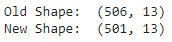My Personal Notes arrow_drop_up